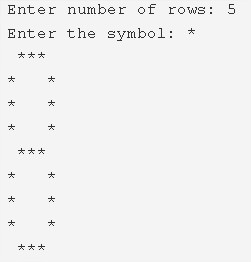# 8 Star Pattern – C Program | 4 Multiple Ways

C Program to print an 8 Star Pattern – In this article, we will detail in on the various ways to print an 8-star pattern in C programming.

Suitable examples and sample programs have also been added so that you can understand the whole thing very clearly. The compiler has also been added with which you can execute it yourself.

The several ways explained in this piece are as follows:

• Using For Loop
• Using While Loop
• Using Do-While Loop

As the name suggests, an 8-Star pattern is a pattern that you have to make with the help of the “*” symbol. It gives out the shape of the mathematical digit 8.

It looks like this:As you can see, you have to first enter the number of rows. Accordingly, your 8-Star pattern will be drawn.

THus, the ways to do the same in C programming are as follows:

## Using For Loop

1) Read and store the entered number of rows and entered a symbol into the variables n, ch.

2) k is defined as k=n*2-1.

3) The outer for loop iterates through rows with the structure for(i=1;i<=k;i++)

4) If( i=1 or i=n or i=k) condition is true then

The 1st inner for loop iterates through columns with the structure for(j=1;j<=n;j++).

a) If(j=1 or j=n) is true then it prints space.

b) Otherwise, it prints symbol.

5) If (i=1 or i=n or i=k)  is false then

The 2nd inner for loop iterates through columns with the structure for(j=1;j<=n;j++).

a) If( j=1 or j=n) the condition is true then it prints symbol.

b) Otherwise, it prints space.

6) Cursor comes to next line for each iteration of outer for loop.

Output:

### Using While Loop

1) k=n*2-1.

2) i=1, The outer while loop with the condition i<=k iterates through rows until the condition becomes false.

3) If (i=1 or i=n or i=k ) condition is true

a) The inner while loop iterates through columns until the condition j<=n becomes false.

It prints space if( j=1 or j=n)is true. Otherwise, it prints symbol.

j value increased by 1.

4) If(i=1 or i=n or i=k)condition is false

a) The 2nd inner while loop iterates through columns until the condition j<=n becomes false.

It prints symbol if(j=1 or j=n)is true.Otherwise, it prints space.

5) The cursor comes to next line.i value increased by 1 of the outer while loop.

Output:

#### Using Do-While Loop

1) k=n*2-1.

2) i=1, The outer do-while loop iterates through rows.

3) If (j=1 or j=n or j=k) condition is true then

j=1, the inner do-while loop iterates through columns.

a) It prints space if j=1 or n.Otherwise, it prints symbol.

b) j value increased by 1.

Checks the condition j<=n.If the condition is true then inner loop iterates again.Repeats until the condition becomes false.

4) If(j=1 or j=n or j=k)condition is false then

j=1, the 2nd inner do-while loop iterates through columns.

a) It prints symbol if j=1 or n.Otherwise, it prints space.

b) j value increased by 1.

Checks the condition j<=n.If the condition is true then 2nd inner do-while loop iterates again until the condition becomes false.

5) The cursor comes to next line. i value increased by 1.

Checks the condition i<=k.If the condition is true then outer loop iterates again.Repeats until the condition becomes false.

Output:
x

## C Program To Print Number Of Days In A Month | Java Tutoring

C program to input the month number and print the number of days in that ...